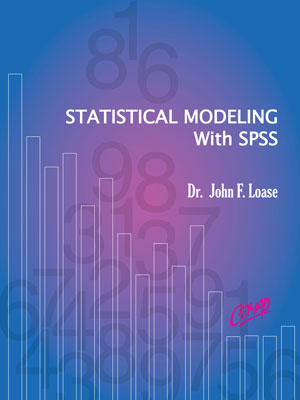| ©2015 by Dr. John F. Loase, Concordia College, New York | Textbook, 505 pages |

Hypothesis testing, confidence intervals, regression and correlation. Using SPSS to analyze large data set.

Random variables, density functions, distribution functions.

Goodness of fit tests, Poisson, normal, uniform density functions. Using SPSS to test data for goodness of fit to key density functions.

Linear congruential generators, empirical tests.

Inverse transform, uniform, exponential densities. Statistical modeling examples with SPSS.